Author: Oscar Cronquist Article last updated on May 31, 2022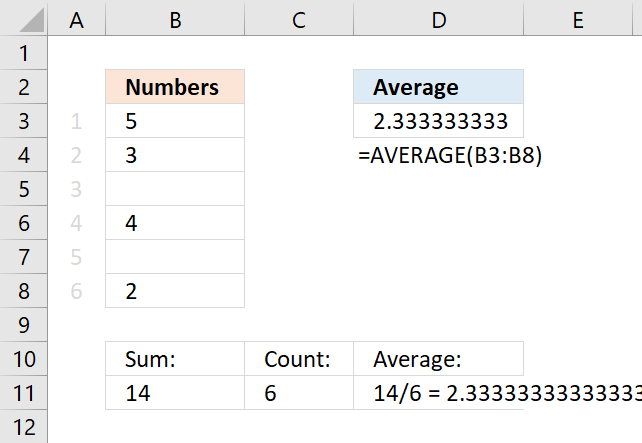## 1. AVERAGE ignore blanksThe AVERAGE function is designed to ignore blank cells automatically but there are instances where it fails. The picture above seems to have blank cells in B3:B8, however, they are counted as zeros. Why?

### 1.1 Cells containing 0 (zero) formatted as a blank

The cells in B3:B8 are not truly empty, select B3:B8 and press CTRL + 1 to open the "Format Cells" settings.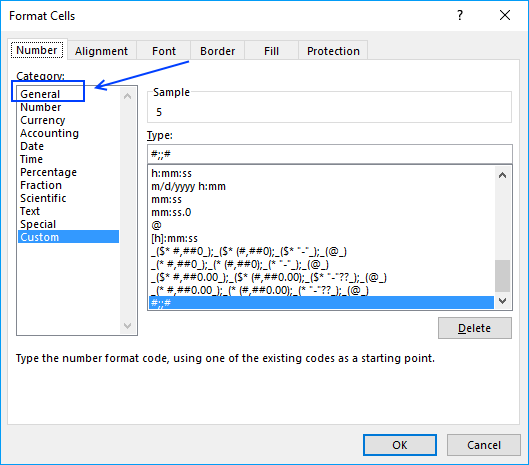Here we see that it has been formatted with custom cell formatting. Values containing 0 (zeros) are hidden, to show them press with left mouse button on General and then OK.

This will show your zeros again on your worksheet, if not read the next section. If you are interested in learning more about custom formatting codes, read this article: Excel’s TEXT function explained

### 1.2 Check your Excel settings

If this didn't do it, go to your Excel Options and then press with left mouse button on "Advanced".

Excel 2016 Users press with left mouse button on "File" on the ribbon and then press with left mouse button on "Options".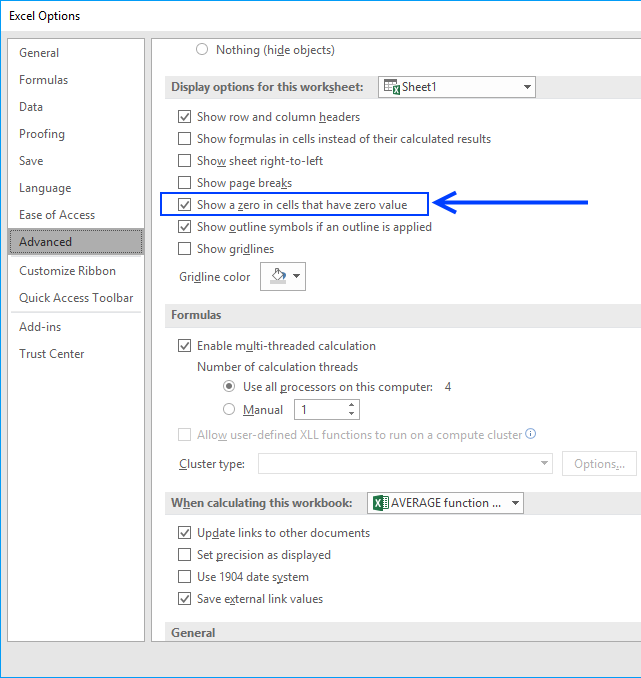Find "Show a zero in cells that have zero value", is it enabled?

## 2. Average - ignore blanks and errors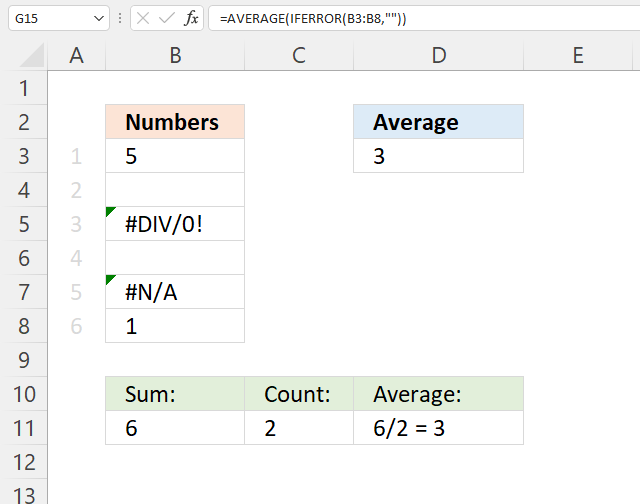The AVERAGE function ignores empty cells out of the box automatically, no need to worry about that. However, if you are working with possible error values and use the IFERROR function to handle errors strange things happen.

The IFERROR function removes errors but has this side effect that it converts blank cells to zeros which in turn makes the AVERAGE function return an incorrect result.

Try this formula: =AVERAGE(IFERROR(B3:B8, "")) it returns 2 if you use the example values above and that is wrong. Use instead the following formula.

Formula in cell D3:

=AVERAGE(IFERROR(IF(B3:B8<>"",B3:B8,NA()),""))

The formula above converts blank cells to error value #N/A! and then remove the error values, here are the steps in detail:

### Explaining formula

#### Step 1 - Identify nonempty cells

The less than and the greater than signs combined evaluate to "not equal to", in this case, not equal to nothing (blank). The result is a boolean value TRUE or FALSE.

B3:B8<>""'

becomes

{5; 0; #DIV/0!; ""; #N/A; 1}<>""

and returns

{TRUE; TRUE; #DIV/0!; FALSE; #N/A; TRUE}.

#### Step 2 - Replace empty values with an error value

The IF function returns one value if the logical test is TRUE and another value if the logical test is FALSE.

IF(logical_test, [value_if_true], [value_if_false])

The IF function replaces TRUE with the corresponding value and FALSE with an error value. Error values in the logical test expression return the same in the IF function.

IF(B3:B8<>"", B3:B8, NA())

becomes

IF({TRUE; TRUE; #DIV/0!; FALSE; #N/A; TRUE}, B3:B8, NA())

and returns

{5; #N/A; #DIV/0!; #N/A; #N/A; 1},

#### Step 3 - Handle error values

The IFERROR function replaces error values with a given value that you specify.

IFERROR(valuevalue_if_error)

In this case it replaces error values with nothing (blank).

IFERROR(IF(B3:B8<>"",B3:B8,NA()),"")

becomes

IFERROR({5; #N/A; #DIV/0!; #N/A; #N/A; 1},"")

and returns

{5; ""; ""; ""; ""; 1}.

#### Step 4 - Calculate the average

The AVERAGE function calculates the average of a group of numbers, text and blank cells are ignored.

AVERAGE(number1[number2], ...)

AVERAGE(IFERROR(IF(B3:B8<>"",B3:B8,NA()),""))

becomes

AVERAGE({5; ""; ""; ""; ""; 1})

and returns 3 in cell D3. 5 + 1 = 6. 6 / 2 = 3.

## 3. Average - ignore blanks in non-contiguous cells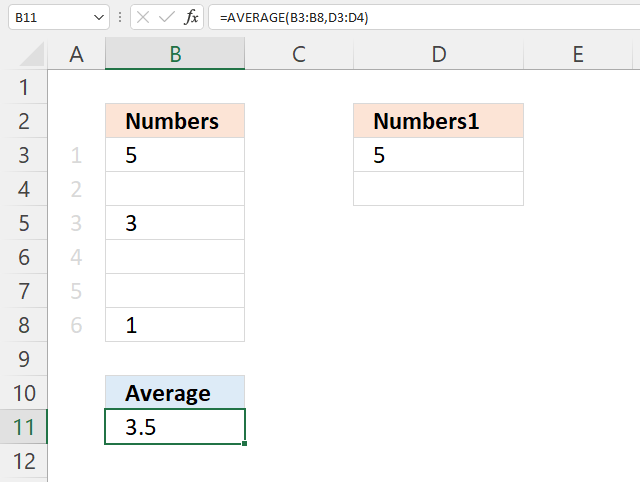The following formula contains two non contiguous cell ranges B3:B8 and D3:D4, the AVERAGE function ignores blank cells automatically. If this is not the case, make sure Excel isn't using a different cell formatting, see section 1.1 and 1.2 above.

Formula in cell B11:

=AVERAGE(B3:B8, D3:D4)

5+3+1+5 = 14. 14/4 = 3.5

## 4. Weighted average ignore blanks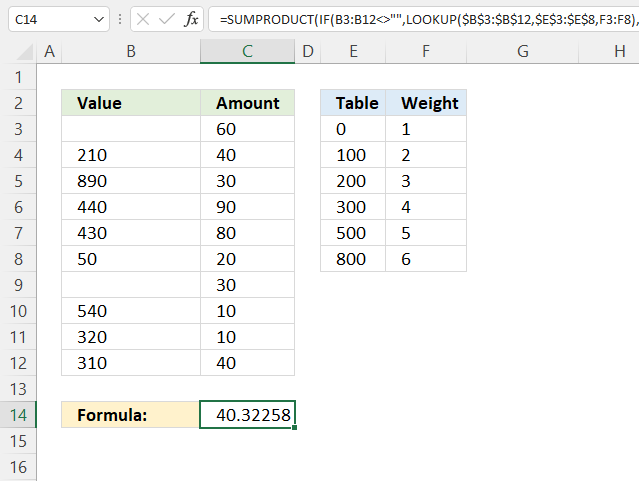Formula in cell C14:

=SUMPRODUCT(IF(B3:B12<>"", LOOKUP(\$B\$3:\$B\$12, \$E\$3:\$E\$8, F3:F8), 0)*C3:C12)/SUM(IF(B3:B12<>"", LOOKUP(\$B\$3:\$B\$12, \$E\$3:\$E\$8, F3:F8), 0))

### 4. Explaining formula in cell C14

#### Step 1 - Find weight based on value

The LOOKUP function lets you find a value in a cell range and return a corresponding value on the same row.

LOOKUP(lookup_valuelookup_vector, [result_vector])

LOOKUP(\$B\$3:\$B\$12, \$E\$3:\$E\$8, F3:F8)

becomes

LOOKUP({0; 210; 890; 440; 430; 50; 0; 540; 320; 310},{0; 100; 200; 300; 500; 800},{1; 2; 3; 4; 5; 6})

and returns

{1; 3; 6; 4; 4; 1; 1; 5; 4; 4}.

#### Step 2 - Replace blank values with 0 (zero)

The IF function returns one value if the logical test is TRUE and another value if the logical test is FALSE.

IF(logical_test, [value_if_true], [value_if_false])

IF(B3:B12<>"", LOOKUP(\$B\$3:\$B\$12, \$E\$3:\$E\$8, F3:F8), 0)

becomes

IF({""; 210; 890; 440; 430; 50; ""; 540; 320; 310}<>"", LOOKUP(\$B\$3:\$B\$12, \$E\$3:\$E\$8, F3:F8), 0)

becomes

IF({FALSE; TRUE; TRUE; TRUE; TRUE; TRUE; FALSE; TRUE; TRUE; TRUE}, LOOKUP(\$B\$3:\$B\$12, \$E\$3:\$E\$8, F3:F8), 0)

becomes

IF({FALSE; TRUE; TRUE; TRUE; TRUE; TRUE; FALSE; TRUE; TRUE; TRUE}, {1; 3; 6; 4; 4; 1; 1; 5; 4; 4}, 0)

and returns {0; 3; 6; 4; 4; 1; 0; 5; 4; 4}.

#### Step 3 - Multiply array with weights

IF(B3:B12<>"", LOOKUP(\$B\$3:\$B\$12, \$E\$3:\$E\$8, F3:F8), 0)*C3:C12

becomes

{0; 3; 6; 4; 4; 1; 0; 5; 4; 4}*C3:C12

becomes

{0; 3; 6; 4; 4; 1; 0; 5; 4; 4}*{60; 40; 30; 90; 80; 20; 30; 10; 10; 40}

and returns

{0; 120; 180; 360; 320; 20; 0; 50; 40; 160}.

#### Step 4 - Add numbers and return a total

The SUMPRODUCT function calculates the product of corresponding values and then returns the sum of each multiplication.

SUMPRODUCT(IF(B3:B12<>"", LOOKUP(\$B\$3:\$B\$12, \$E\$3:\$E\$8, F3:F8), 0)*C3:C12)

becomes

SUMPRODUCT({0; 120; 180; 360; 320; 20; 0; 50; 40; 160})

and returns 1250.

#### Step 5 - Calculate the total weight

The SUM function calculates a sum based on a cell range or array.

SUM(IF(B3:B12<>"", LOOKUP(\$B\$3:\$B\$12, \$E\$3:\$E\$8, F3:F8), 0)

becomes

SUM(IF(B3:B12<>"", {1; 3; 6; 4; 4; 1; 1; 5; 4; 4}, 0)

becomes

SUM({0; 3; 6; 4; 4; 1; 0; 5; 4; 4})

and returns 31.

#### Step 6 - Divide total by total weight

SUMPRODUCT(IF(B3:B12<>"", LOOKUP(\$B\$3:\$B\$12, \$E\$3:\$E\$8, F3:F8), 0)*C3:C12)/SUM(IF(B3:B12<>"", LOOKUP(\$B\$3:\$B\$12, \$E\$3:\$E\$8, F3:F8), 0))

becomes

1250/SUM(IF(B3:B12<>"", LOOKUP(\$B\$3:\$B\$12, \$E\$3:\$E\$8, F3:F8), 0))

becomes

1250/31

and returns 40.3225806451613.

## 5. Average ignore 0 (zero) and blanks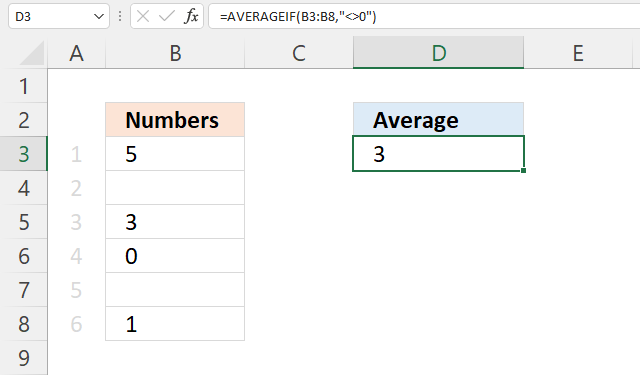The AVERAGE function ignores empty cells automatically. If this is not the case, make sure Excel isn't using a different cell formatting, see section 1.1 and 1.2 above.

Formula in cell B11:

=AVERAGEIF(B3:B8,"<>0")

5+3+1=9. 9/3 = 3